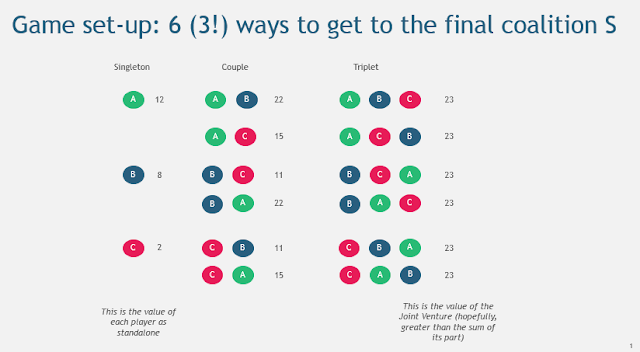### Focus: Shapley value

Game theory is a fascinating topic codifying and quantifying all sorts of interactions between stakeholders in a game. The most popular setup is the prisoner's dilemma but there is much more to it. Today, we will cover the Shapley value as I recently stumbled across this original yet relatively unknown concept.

## Problem at stake:

Example 1: In a salary negotiation, the employee showcases his skills and what it brings to the company. But how much are these skills worth ?
Example 2: In a Joint Venture, each founding company brings expertise. What’s a fair distribution of the ownership/shares ?
Example 3: 2 (or 3) TelCo companies want to build a fiber network that would benefit both. What’s a fair payment breakdown ?

When you think about these problems, most of us would tend to answer them through guesses: “You should ask for X% raise because you deserve it” but there is actually a theory for it

Let’s look at the theory behind.

## Solution:

This is known as a cooperative game and there is only one breakdown function that fulfill a few conditions (more in this later).

The gist of it: “Player A fair reward is the average of his marginal contributions to the different coalitions leading to the final setup“ where:

For a game with 3 players (A,B,C), we denote:
-          Final setup: final set of stakeholders S {A,B,C}
-          Coalition: a subset of S
-          Marginal contribution: Adding A to {A,B} is : Value {A,B,C} – Value {B,C}

Now, let’s introduce value to the example. For instance:
-          V(A) = 12
-          V(B) = 8
-          V(C) = 2
-          V(A,B) = 22
-          V(A,C) = 15
-          V(B,C) = 11
-          V(A,B,C) = 23The Sharpley value of A is calculated as:## Generalization

From there, you can intuit the general formula where n! is the total number of permutations :

Where K \ A notes the coalition K without A.